# midas Structure

BLOG PROJECT TUTORIAL

# Wind Load as per ASCE 7-16

## Information of Example Model

This is an explanation how to input wind load data in the warehouse with gable hip roof(Two-way).

Information of the example model is given as follows.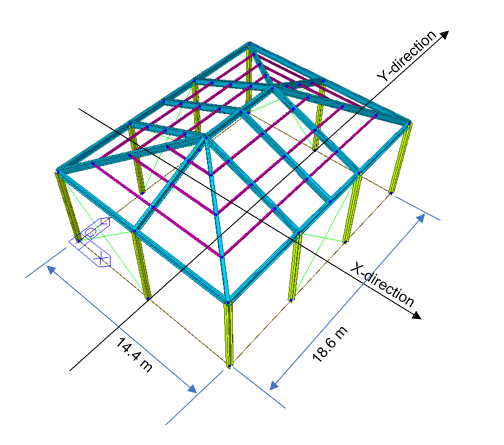Figure1. Warehouse Example Model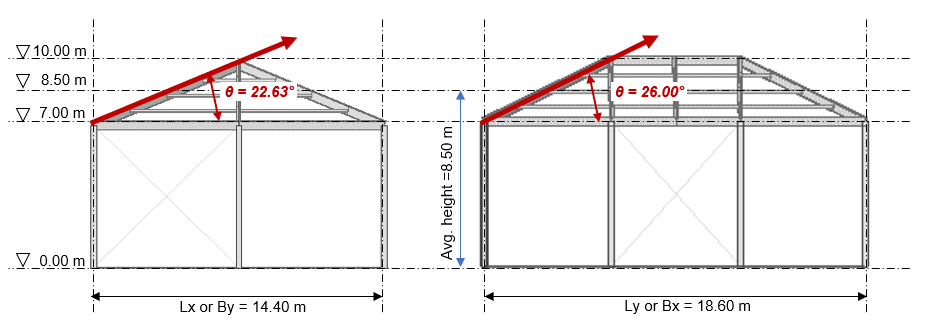Figure 2. Warehouse Roof Example Model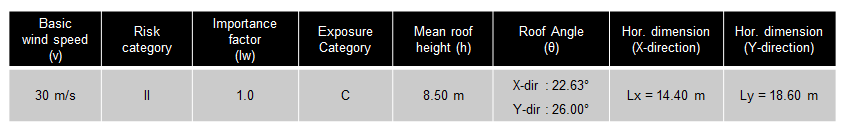Table 1. Wind Load Data Informations

# Calculation of Wind Load - Velocity Pressure

The velocity pressure should be calculated in order to get the value of the wind load.

• Velocity Pressure : qz = 0.613KzKztKdKeV2

1) Determination of Ke (Ground Elevation Factor)

It usually applies ' 1.0 ' on the Ground Elevation Factor, Ke, if there is no exceptional case caused.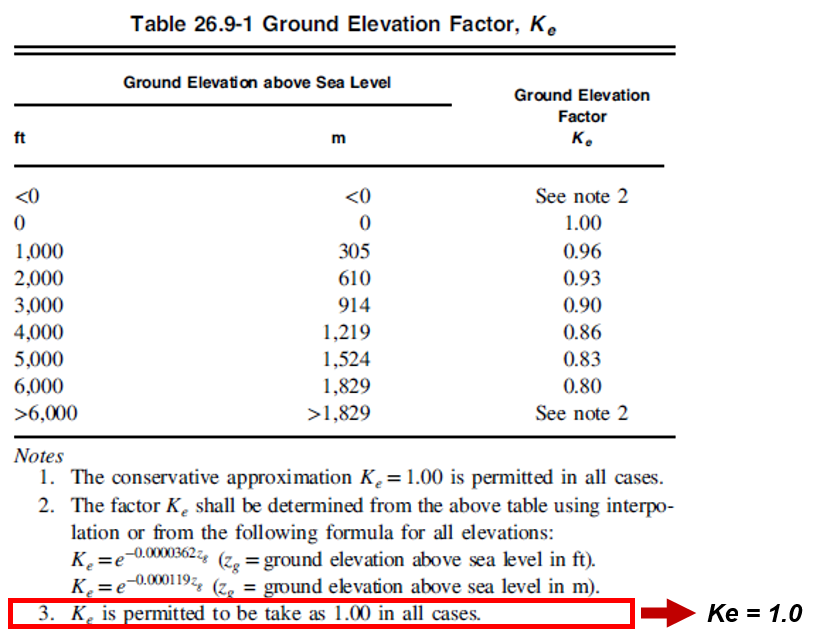Figure 3. Ground Elevation Factor

## 2) Determination of Kd (Wind Directionality Factor)

It applies ' 0.85 ' since the wind directionality factor is based on the structure.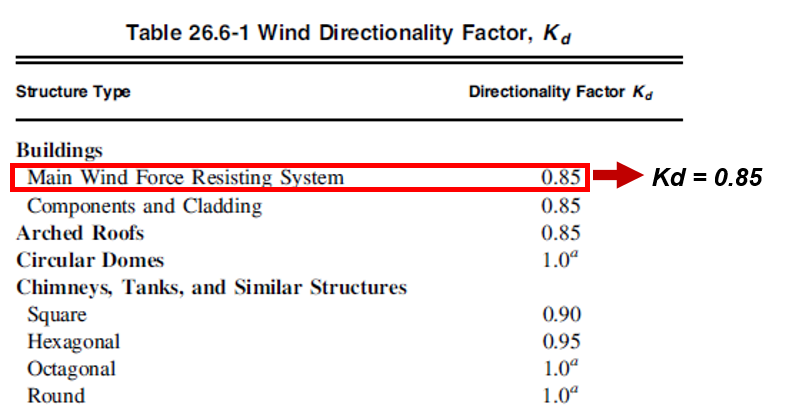Figure 4. Wind Directionality Factor

## 3) Determination of Kz (Velocity Pressure Exposure Coefficient)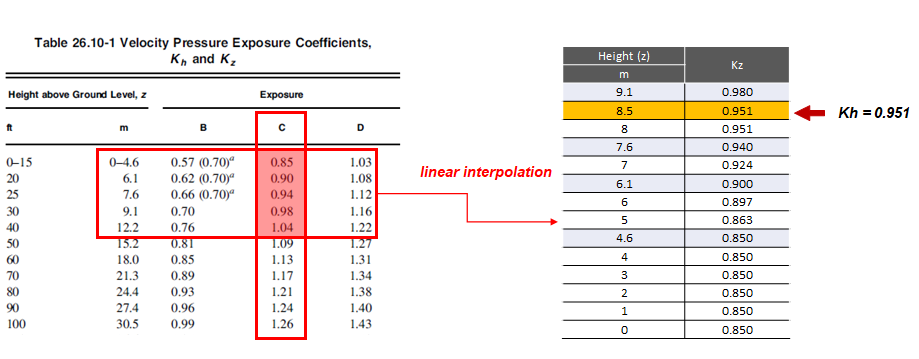Figure 5. Velocity Pressure Exposure Coefficients

## 4) Determination of Kzt (Topographic Effects)

• Kzt = (1+K1K2K3)2

• Assumed ValuesHill Type : 2S Escarpment

H = 20m, Lh = 40m, x = 40m  →  H/Lh = 20/40 = 0.5, x/Lh = 40/40 = 1.0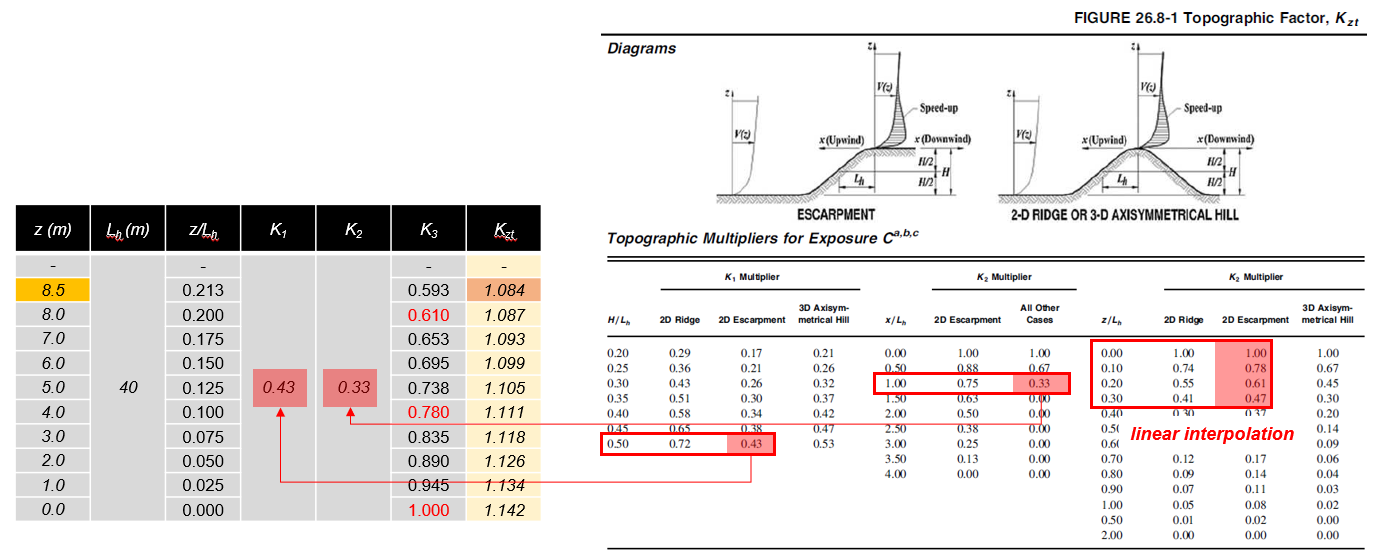Figure 6. Determination of Topographic Effects

## 5) Determination of qz and qh

The following is a table that indicates the final value of the velocity pressure after calculating values for all variables. To calculate wind pressure, qh value for the roof level is applied.

Applying the pressure value depending on levels is more exact, but, it is okay to put the load value by using only the value of qh instead.

• Velocity Pressure : qz = 0.613KzKztKdKeV≈ 490N/㎡ =qh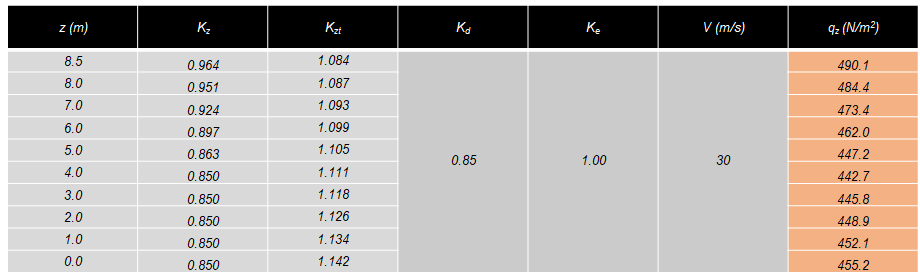Table 2. Velocity Pressure Value

# Calculation of Wind Load - External Wind Pressure

p = qGCP - qi(GCpi)

Case 1) Wind Pressure = External Wind Pressure - Internal Wind Pressure (Positive)

Case 2) Wind Pressure = External Wind Pressure - Internal Wind Pressure (Negative)

Two coefficient values for external pressure and internal pressure are needed in order to satisfy the formula. Basically, wind pressure has two values depending on the direction of internal pressure. If internal pressure is larger than the external pressure, positive internal pressure is generated. In the opposite case, negative internal pressure is generated.

Figure 7. Positive Internal Wind Pressure and Negative Internal Wind Pressure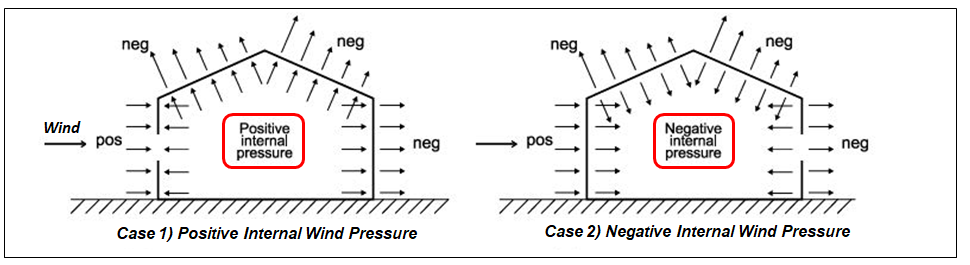## 1) External Wind Pressure for Gable Roof Direction

Wind ward wall, leeward wall, and side wall are needed to satisfy the calculation of external wind pressure.

The following is an explanation of the calculation for the x-direction and wall.

• Gust Factor (G) = 0.85 (Assumed Value)

* You can get the gust factor as per 26. 11. 4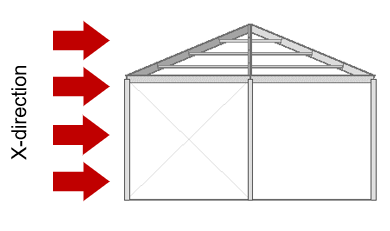Figure 8. External Wind Pressure in X-Direction

• Wall Pressure Coefficients (Cp)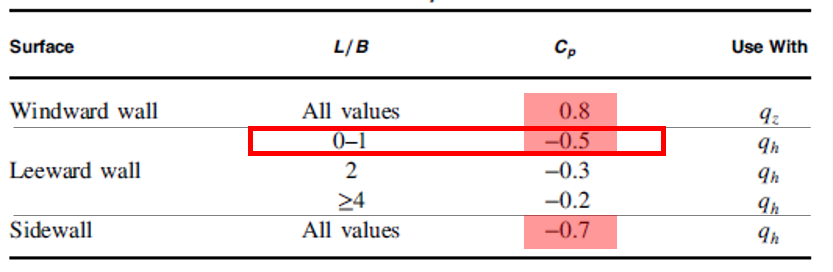Figure 9. Wall Pressure Coefficients - 1

* L/B =14.4/ 18.6 = 0.774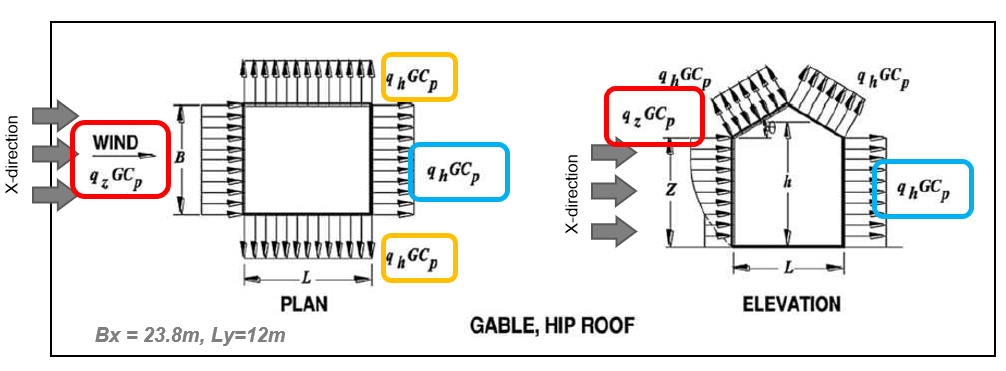Figure 10. Wall Pressure Coefficients - 2

* Bx = 23.8m, Ly = 12m → L/B = 0.504

Red Box : Wind load for windward wall (Wx(+ or -)_Windward)

q_wind = qzGCP = 490 * 0.85 * 0.8 = 333.2 N/m2

Blue Box : Wind load for Leeward wall (Wx(+ or -)_Leeward)

q_lee = qhGCP = 490 * 0.85 * - 0.5 = -208.3 N/m2

Yellow Box : Wind load for Side wall (Wx(+ or -)_Side)

q_side = qhGCP = 490 * 0.85 * - 0.7 = -291.6 N/m2

The following is an example of the calculation for the roof in X-Direction.

• Gust Factor (G) = 0.85 (Assumed Value)

* You can get the gust factor as per 26. 11. 4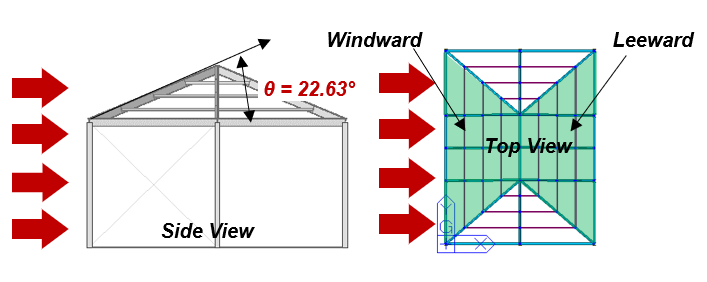Figure 11. External Wind Pressure for the Roof in X-Direction

• Roof Pressure Coefficients, Cp, for use  with qh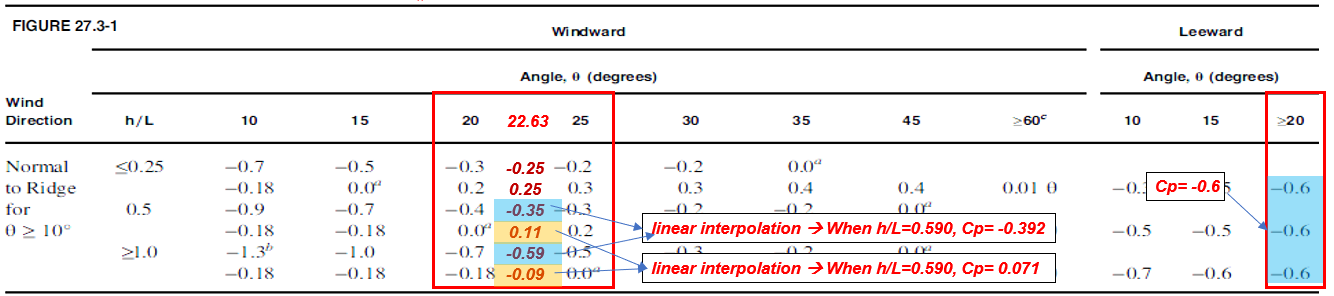Figure 12. Roof Pressure Coeffocoents - 1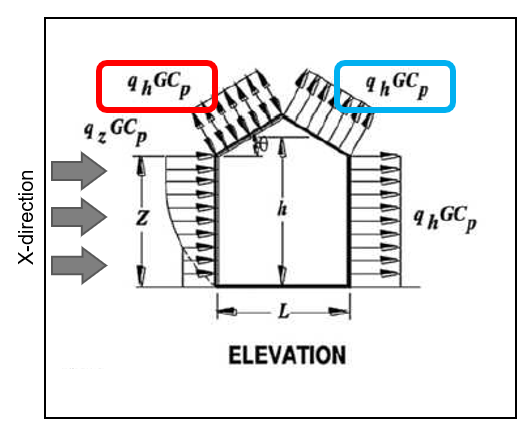Figure 13. Roof Pressure Coeffocoents - 2

* h = 8.5m, Ly =14.4m → h/L = 0.590

Red Box : Wind load for windward roof (Wx(+ or -)_Windward)

q_wind = qzGCP = 490 * 0.85 * -0.392 = -163.27 N/m2

q_wind = qzGCP = 490 * 0.85 * -0.071 = -29.58 N/m2

Blue Box : Wind load for Leeward wall (Wx(+ or -)_Leeward)

q_lee = qhGCP =490 * 0.85 * - 0.6 = -249.94N/m2

The following is an example of the calculation for the roof in X-Direction for the side area.

• Gust Factor (G) = 0.85 (Assumed Value)

* You can get the gust factor as per 26. 11. 4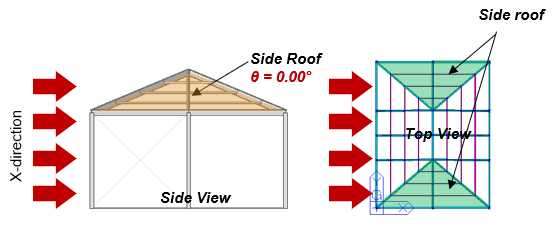Figure 14. External Wind Pressure for the Side Roof in X-Direction

• Roof Pressure Coefficients, Cp, for use  with qh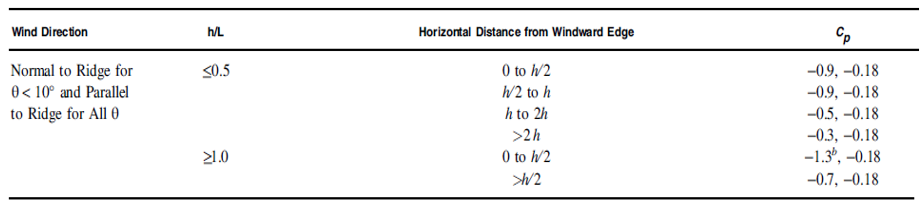Figure 15. Side Roof Pressure Coeffocoents - 1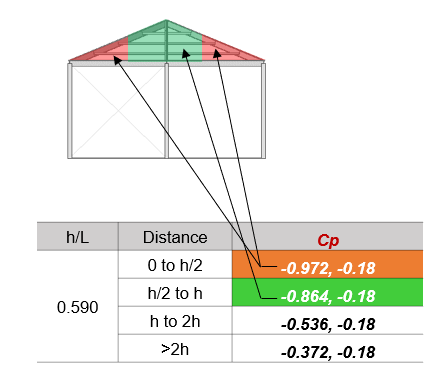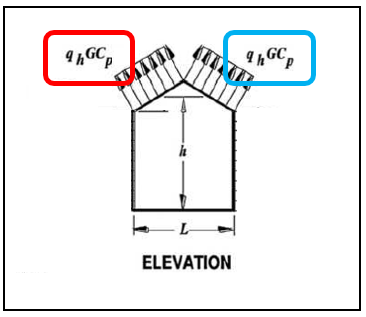Figure 16.Side Roof Pressure Coeffocoents - 2

* h = 8.5m, Ly =14.4m → h/L = 0.590

Red Box : Wind load for windward roof (Wx(+ or -)_Windward)

q_wind = qzGCP = 490 * 0.85 * -0.972(Apply Max.Cp)= -404.9 N/m2

q_wind = qzGCP = 490 * 0.85 * -0.18= -75.0 N/m2

Blue Box : Wind load for Leeward wall (Wx(+ or -)_Leeward)

q_lee = qhGCP =490 * 0.85 * - 0.6 = -249.94N/m2

## 2) External Wind Pressure for Gable Roof Orthogonal Direction

The following is an explanation of the calculation for the y-direction. The wall pressure for the y-direction can be calculated as the x-direction way.

• Gust Factor (G) = 0.85 (Assumed Value)

* You can get the gust factor as per 26. 11. 4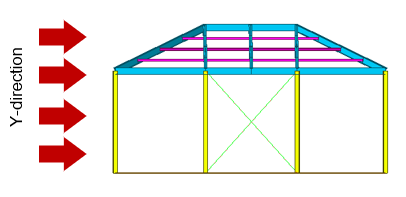Figure 17. External Wind Pressure in Y-Direction

• Wall Pressure Coefficients (Cp)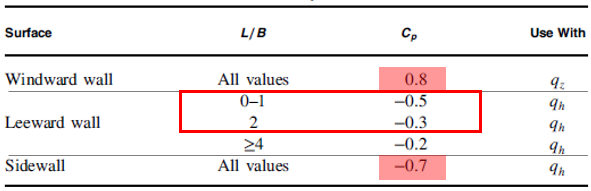Figure 18. Wall Pressure Coefficients - 1
* L/B =18.6/ 14.4 = 1.29  (Cp=-0.42)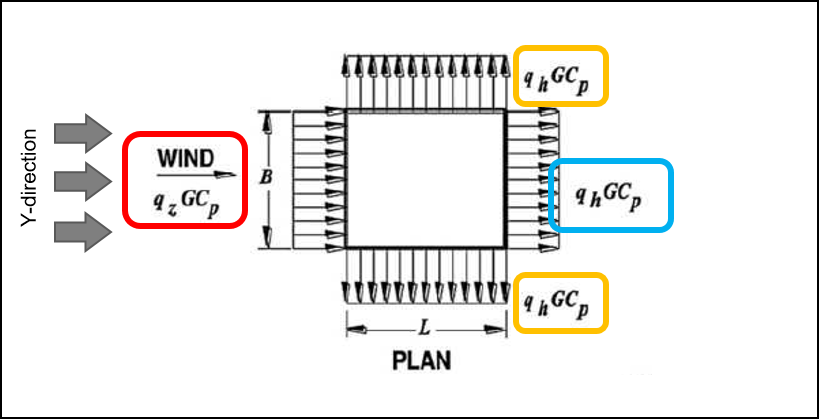Figure 19. Wall Pressure Coefficients - 2

* By = 14.4m, Lx =18.6m → L/B = 1.29

Red Box : Wind load for windward wall

q_wind = qzGCP = 490* 0.85 * 0.8 = 340 N/m2

Blue Box : Wind load for Leeward wall

q_lee = qhGCP = 500 * 0.85 * -0.3 = -127.5N/m2

Yellow Box : Wind load for Side wall

q_side = qhGCP = 500* 0.85 * -0.7 = -297.5N/m2

The following is an example of the calculation for the roof in Y-Direction.

• Gust Factor (G) = 0.85 (Assumed Value)

* You can get the gust factor as per 26. 11. 4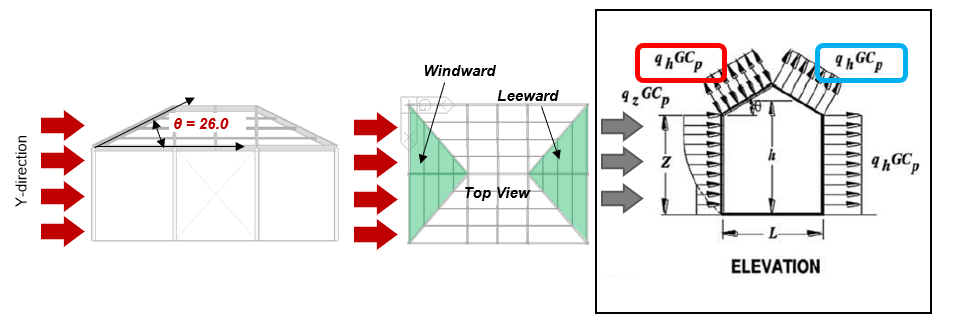Figure 20. External Wind Load in Y-Direction and Angle

* h = 8.5m, Lx = 18.6m → h/L = 0.457

Red Box : Wind load for windward roof (Wx(+ or -)_Windward)

q_wind = qzGCP = 490 * 0.85 * -0.266 = -110.81N/m2

q_wind = qzGCP = 490 * 0.85 * -0.217 = -90.39N/m2

Blue Box :Wind load for leeward roof (Wx(+ or -)_Leeward)

q_lee = qhGCP = 490 * 0.85 * -0.6 = -249.94N/m2

• Roof Pressure Coefficients, Cp, for use with qh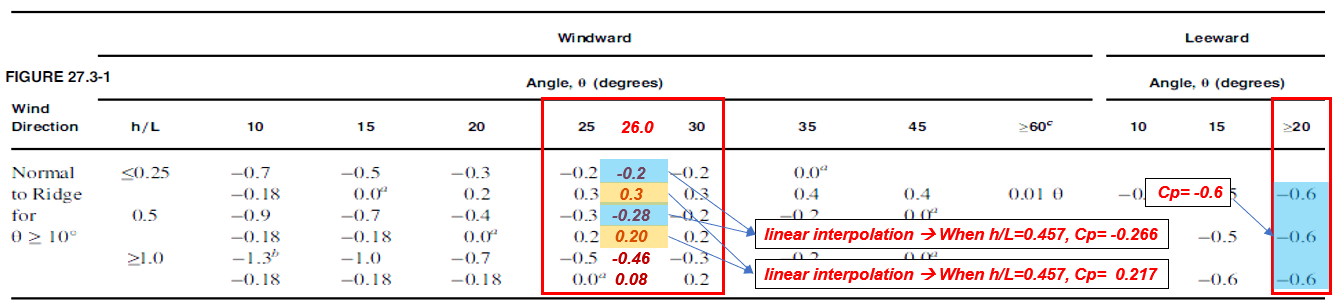Figure 21. Roof Pressure Coefficients

The following is an example of the calculation for the roof in Y-Direction for the side area.

• Gust Factor (G) = 0.85 (Assumed Value)

* You can get the gust factor as per 26. 11. 4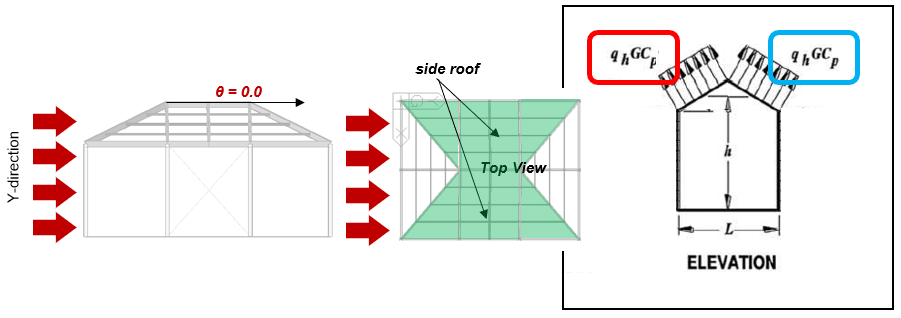Figure 22. External Wind Load for Side Roof in Y-Direction and Angle

* h = 8.5m, Lx = 18.6m → h/L = 0.457

Red Box : Wind load for windward roof (Wx(+ or -)_Windward)

q_wind = qzGCP = 490 * 0.85 * -0.9 = -374.90N/m2

q_wind = qzGCP = 490 * 0.85 * -0.18 = -74.98N/m2

• Roof Pressure Coefficients, Cp, for use with qh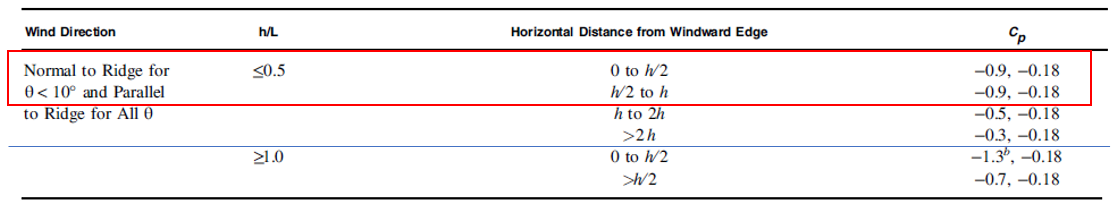Figure 23. Side Roof Pressure Coefficients

# Calculation of Wind Load - Internal Wind Pressure

## 1) Internal Wind Pressure for Gable Roof Orthogonal Direction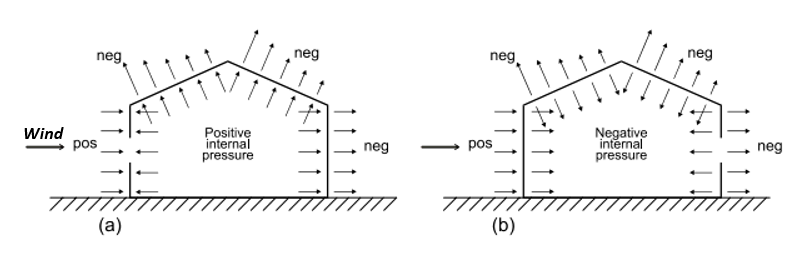Figure 24 Internal Wind Pressure for Gable Roof Orthogonal Direction

Partially enclosed buildings in enclosure classification would be applied in this example. Therefore, the value of the internal pressure coefficient can be assumed as +0.55 and -0.55.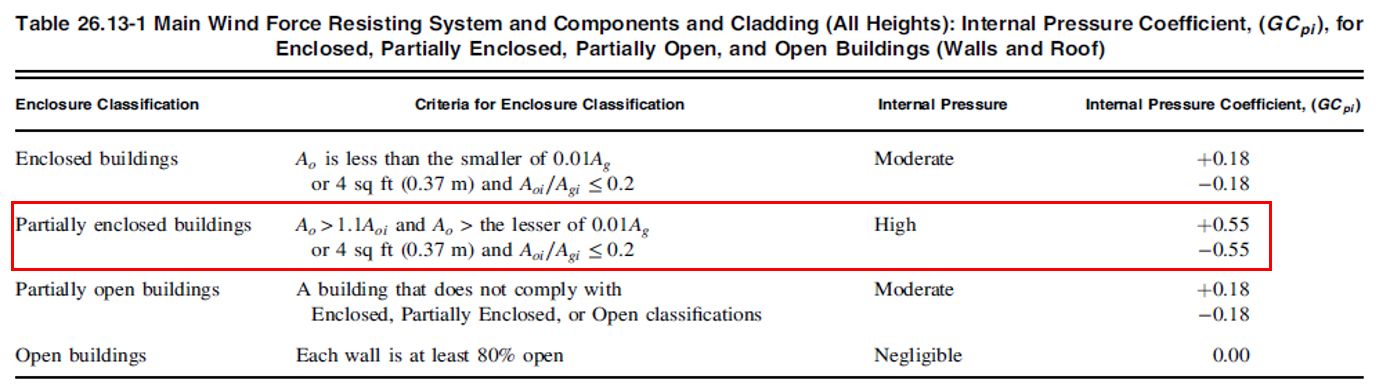Figure 25. Table for Internal Pressure Coefficient

## 2) Wind Pressure on Wall for Gable Roof Direction (along x-direction)

Wind pressure in the x-direction is given as follows. The values of each case in the wind pressure are calculated by applying the values of the external pressure and internal pressure.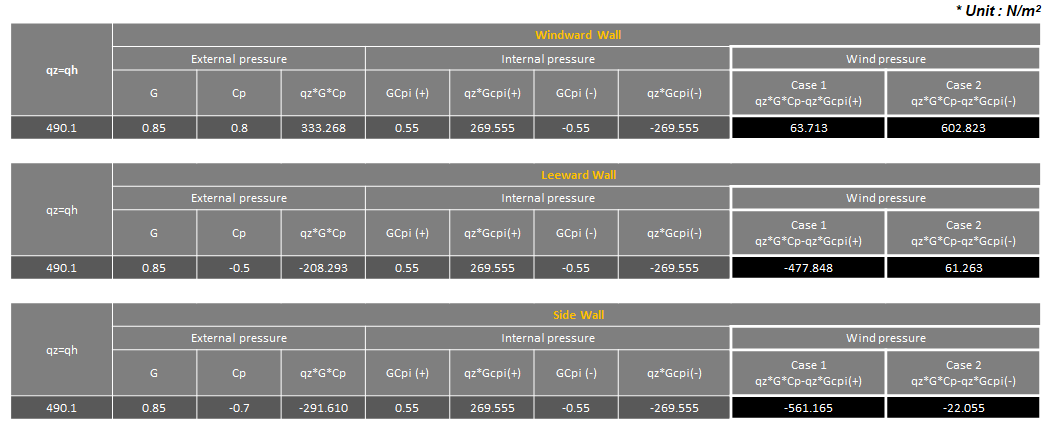Table 3. Wall Wind Pressure Values along X-Direction

## 3) Wind Pressure on Roof for Gable Hip Roof Direction (along x-direction)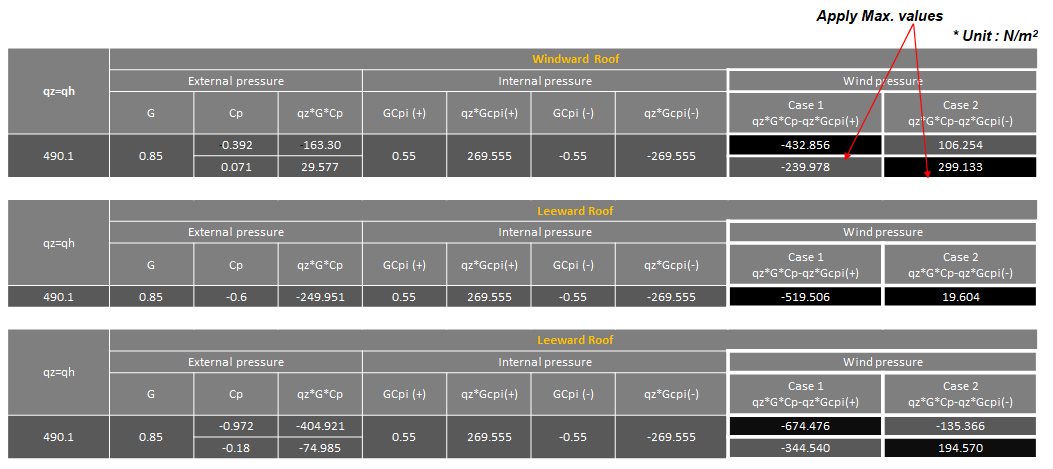Table 4. Roof Wind Pressure Values along X-Direction

## 4) Wind Pressure on Wall for Gable Hip Roof Orthogonal Direction (along y-direction)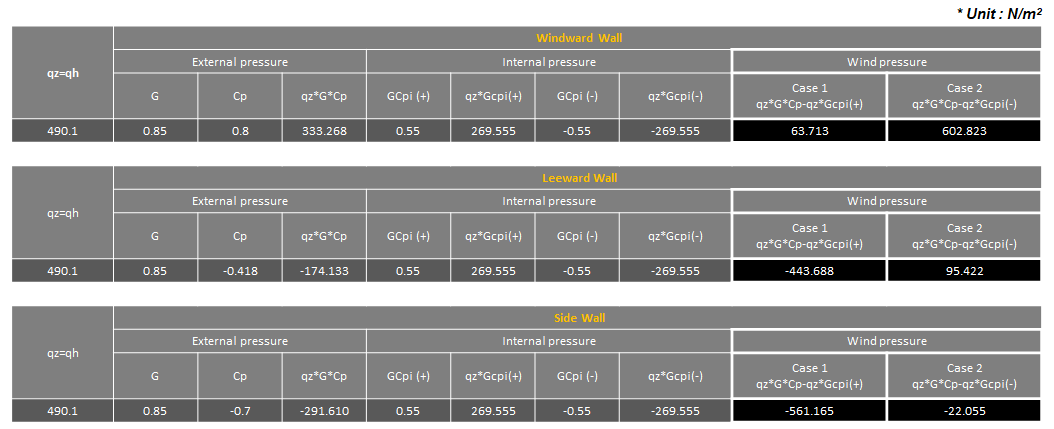Table 5. Wall Wind Pressure Values along Y-Direction

## 5) Wind Pressure on Roof for Gable Roof Orthogonal Direction (along y-direction)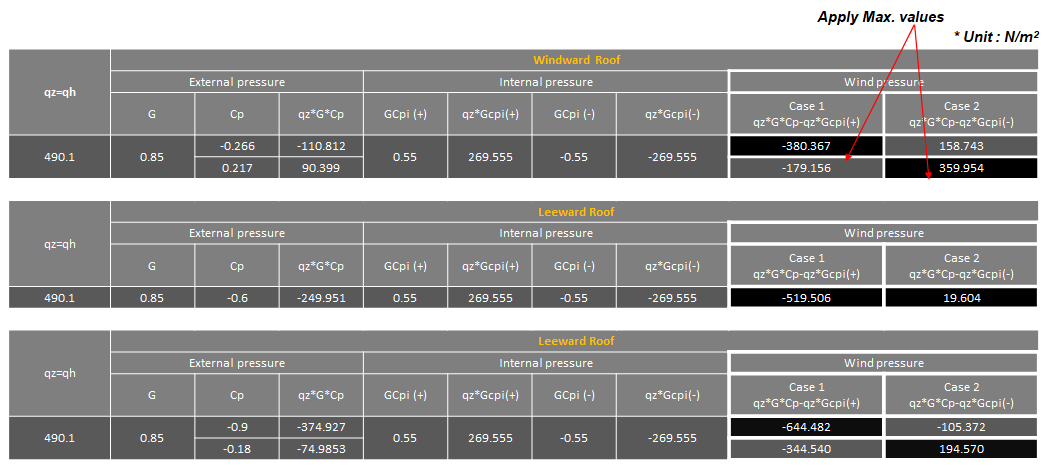Table 6.  Roof Wind Pressure Values along Y-Direction

# Wind Pressure Summary

The final values for cases 1 & 2 are as follows.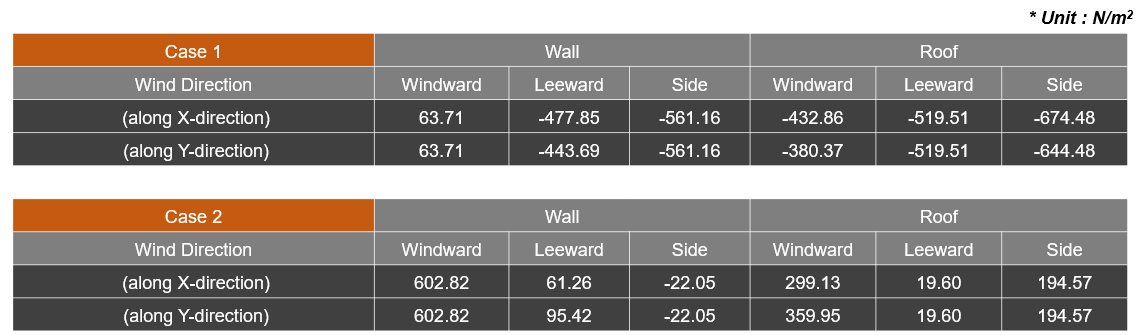Table 7. Values for Cases 1 and 2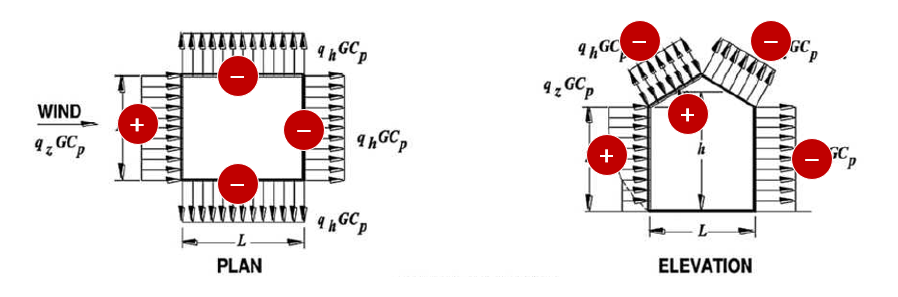Figure 26. Direction Sign of the Building

• Sign (+) : Direction to the inside of the building

• Sign (-) : Direction to the outside of the building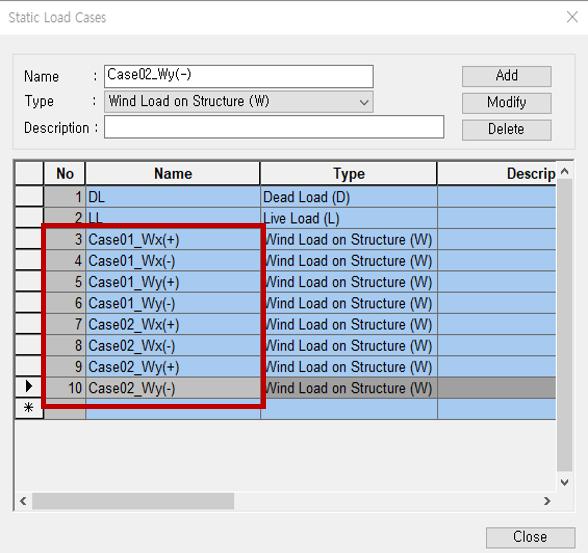Figure 27. Static Load Cases Dialog Box

* Tips : you can easily distinguish each case if you put the name above.

Considered Factors

- Case : Case 1 & Case 2

- Wind Direction : X-Direction & Y- Direction

- Wind Sign : (+) & (-)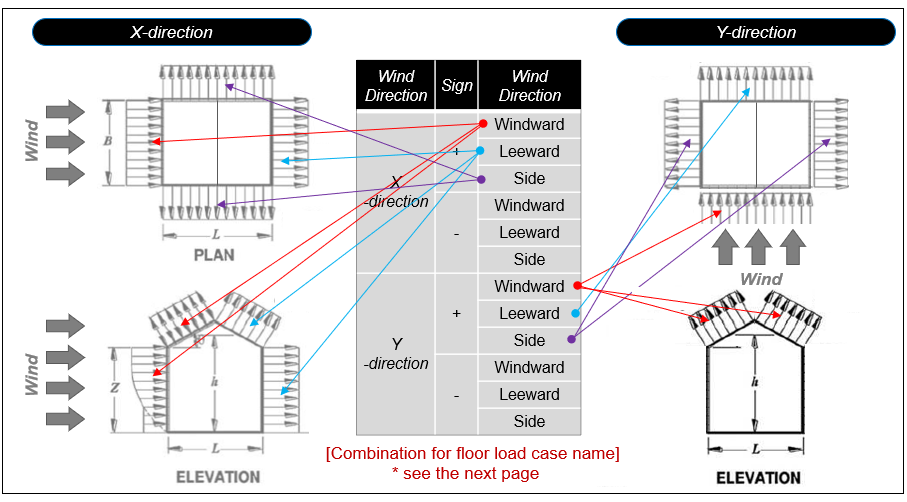Figure 28. Information of Floor Load Cases for Wind Load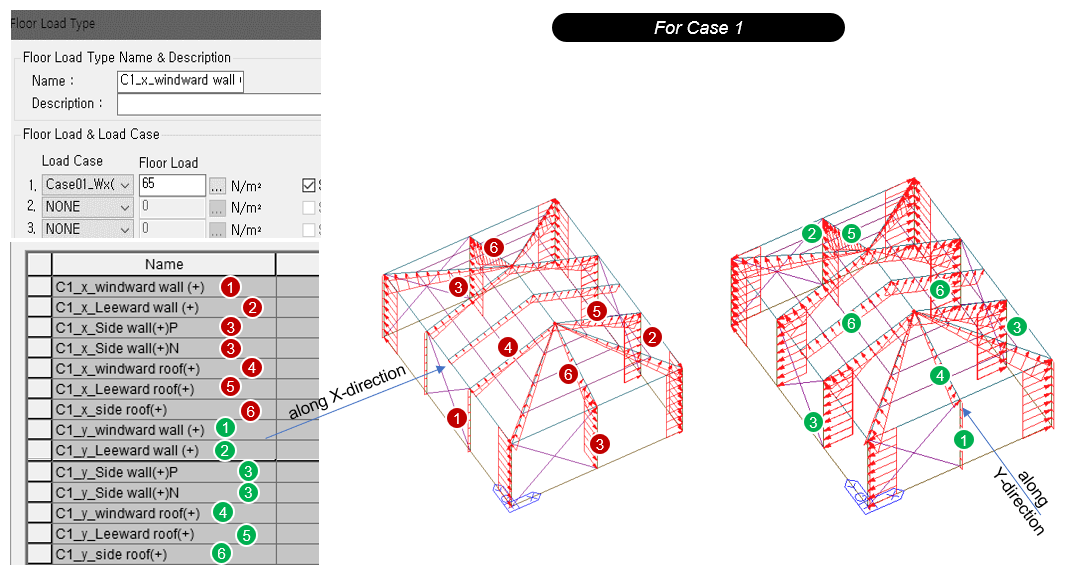Figure 29. Floor Load Type Dialog Box for Case 1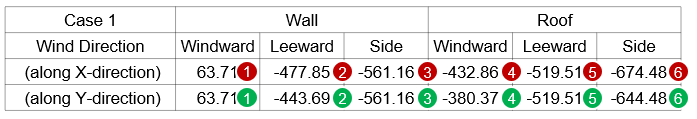Table 8. Case 1 Values of Wall and Roof

• Sign (+) : Direction to the inside of the building

• Sign (-) : Direction to the outside of the building

When inputting the wind load into the model,  the sign must be corrected according to the direction of the load.

It also creates a floor load type for the opposite direction.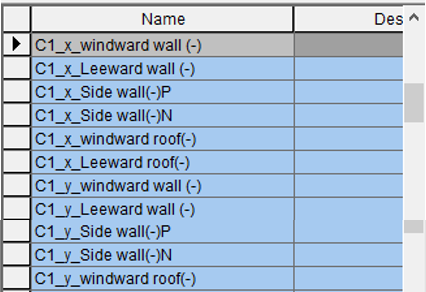Figure 30. Case 1 Name List Dialog Box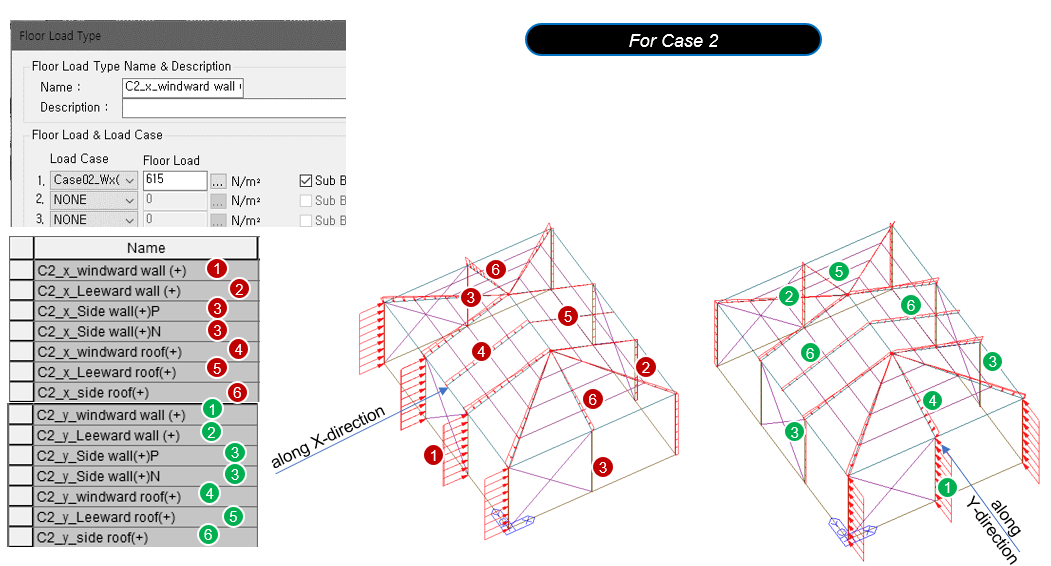Figure 31. Floor Load Type Dialog Box for Case 2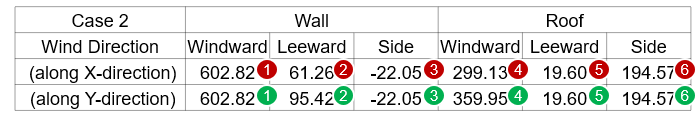Table 9. Case 2 Values for Wall and Roof

• Sign (+) : Direction to the inside of the building

• Sign (-) : Direction to the outside of the building

When inputting the wind load into the model,  the sign must be corrected according to the direction of the load.

It also creates a floor load type for the opposite direction.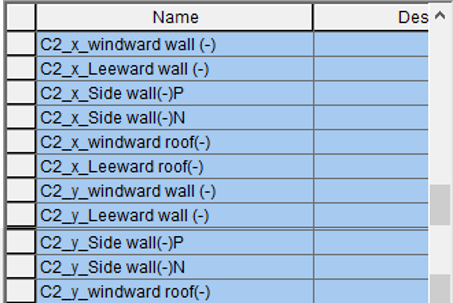Figure 32. Case 2 Name List Dialog Box

Before performing analysis and design, we recommend you make load combinations with a wind load. Even if it is not automatically generated, you can easily create it by using a table for the load combination or copying it from excel.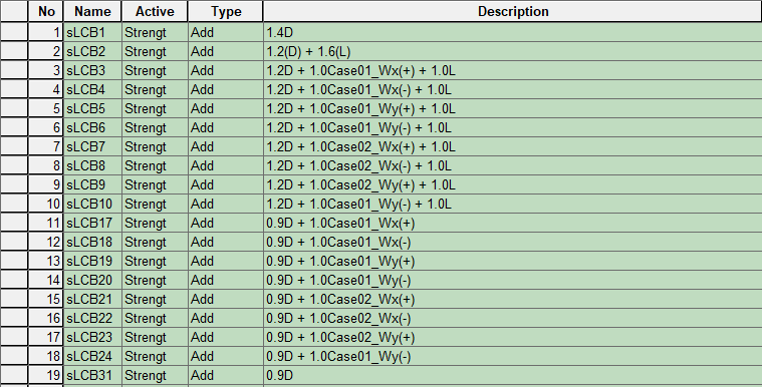Figure 33. Load Combination Example - 1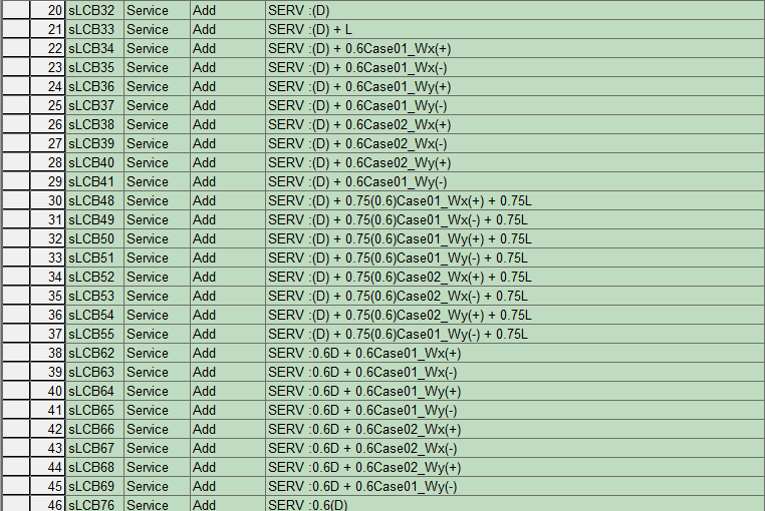Figure 34. Load Combination Example - 2

Yeong-il SeoYeong-il Seo | Principal Structural Engineer

Young-il has over 13+ years of experience in building design, especially high-rise buildings with column reduction analysis, plant structures, pushover analysis, health monitoring, and vibration control projects. Since 2016, he is planning and providing technical supports for midas building products such as midas Gen, nGen, and Design+.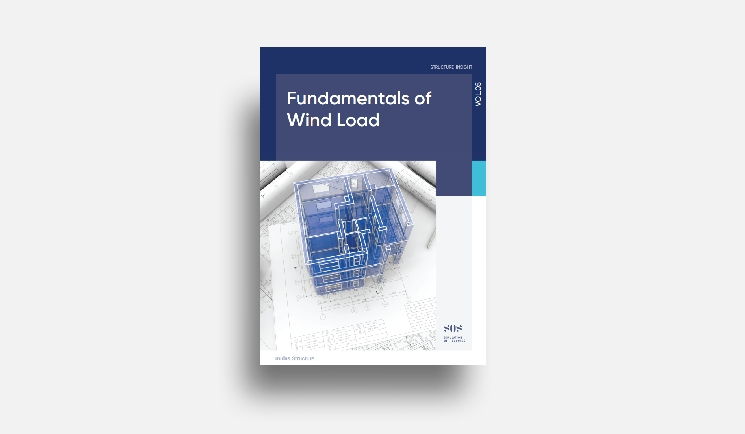E-BOOK Fundamentals of